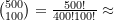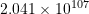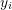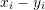# The Optimal Tradeoff Between Diversification and Outperformance

Warren Buffet once said that, “diversification is protection against ignorance”. Of course, the other side is true as well: strategies that aren’t adequately diversified run a substantially higher risk of ruin. The goal of this paper is to find a useful approximation for portfolio size that a. increases the potential for alpha (return over benchmark) at a constraint of b. minimal beta (volatility relative to benchmark).

To explore this question, we look at S&P 500 stocks over the last three calendar years and run repetitive randomized simulations. We use the full equal-weighted set of stocks as our benchmark. Each trial in our simulation randomizes the selection of 100 stocks, placing no bias on size or industry. It is impossible to examine thepossible unique portfolios of 100 stocks, so we instead elect to run 500 trials, plenty enough to show general trends.

Figure 1 below shows the returns of the five hundred portfolios next to the equal-weighted index (the thick black line). Each colored line in the figure represents a different portfolio; the left-most part shows the annualized return of a single stock while the right-most part of the same line shows the annualized return of the stock and 99 others all held in tandem at 1% weight. Notice how the potential to meaningfully over/underperform the index exists largely in portfolios with less than about 40 stocks—much more than that and an active strategy is at risk of becoming a “closet indexer”. For perspective, 11.60% of the portfolios generated more than 5% alpha, of which 69.73% had less than 40 stocks.

## Figure 1: Annualized Return of Random Buy-and-Hold Strategies

It’s intuitive that standard deviation and the number of portfolio holdings move in inverse. This intuition is borne out by the data as shown in Figure 2 below– there is a clear exponential relationship between volatility and the number of portfolio holdings (the benefit of increasing from 1 stock to 10 stocks is much larger than the benefit of increasing from 91 stocks to 100, for example). Due to this exponential relationship, any more than about 30 stocks has a negligible effect on standard deviation.

## Figure 2: Annualized Standard Deviation of Random Buy-and-Hold Strategies

Using these two pieces of information, we can try to strike a balance between returns and volatility. To do so, we elect to examine alpha potential relative to the risk of ruin. Letdenote the upper quartile of returns for a portfolio withstocks and letdenote the maximum drawdown for the same portfolio. For a fair comparison, we normalize both data sets and give equal weight to each variable. We are therefore looking to maximize the value. Figure 3 below shows that this value is maximized when the size of the portfolio is between about 20 and 40 stocks.

## Figure 3: Evaluating Return Potential Relative To Risk

Here at Julex, we design portfolios that strike a balance between outperformance and risk. For example, Julex’s TrueAlphaTM Small Cap Strategy holds no more than 40 stocks at a time (and often has 90% or more concentration in 20 stocks). Of course, unlike the simulation, Julex’s Small Cap portfolio is chosen based on an assortment of factors rather than randomly. By concentrating portfolio holdings to no more than 40 names, Julex can exploit their selection edge, which has led to consistent outperformance over the Russell 2000 index (see Figure 4 below) and a five-star rating from Morningstar.